# Java入门系列之集合ArrayList源码分析

## ArrayList源码分析

  ArrayList<Integer> list = new ArrayList<>();
list.add(1);

### 初始化容量分析

public ArrayList(int initialCapacity) {
if (initialCapacity > 0) {
this.elementData = new Object[initialCapacity];
} else if (initialCapacity == 0) {
this.elementData = EMPTY_ELEMENTDATA;
} else {
throw new IllegalArgumentException("Illegal Capacity: "+
initialCapacity);
}
}

public ArrayList() {
this.elementData = DEFAULTCAPACITY_EMPTY_ELEMENTDATA;
}
.....

    //默认初始化容量
private static final int DEFAULT_CAPACITY = 10;

//数组空实例
private static final Object[] EMPTY_ELEMENTDATA = {};

//默认空数组实例
private static final Object[] DEFAULTCAPACITY_EMPTY_ELEMENTDATA = {};

//被操作数组
transient Object[] elementData;

//数组大小
private int size;

//数组最大容量
private static final int MAX_ARRAY_SIZE = Integer.MAX_VALUE - 8;

### 添加元素分析

//添加元素实现
ensureCapacityInternal(size + 1);
elementData[size++] = e;
return true;
}

private void ensureCapacityInternal(int minCapacity) {
ensureExplicitCapacity(calculateCapacity(elementData, minCapacity));
}
    //计算容量
private static int calculateCapacity(Object[] elementData, int minCapacity) {
//当实例化集合时未给定数组容量或者指定容量为0时，则此时数组为空数组实例
if (elementData == DEFAULTCAPACITY_EMPTY_ELEMENTDATA) {
//此时minCapacity为1，通过Math.max函数将minCapacity和DEFAULT_CAPACITY(默认容量)比较返回【10】
return Math.max(DEFAULT_CAPACITY, minCapacity);
}
//当实例化时给定数组容量大于0，则直接返回添加一个元素后的容量即（size+1）
return minCapacity;
}
    //判断是否扩容
private void ensureExplicitCapacity(int minCapacity) {
modCount++;

// 若计算过后的数组容量大于数组存储长度时则扩容
if (minCapacity - elementData.length > 0)
grow(minCapacity);
}
//扩容核心实现
private void grow(int minCapacity) {

//被操作数组实际容量
int oldCapacity = elementData.length;

//新容量 = （实际容量 + 实际容量/2并去模）即1.5倍旧容量
int newCapacity = oldCapacity + (oldCapacity >> 1);

//若新容量小于数组大小则以数组大小为新容量
if (newCapacity - minCapacity < 0)
newCapacity = minCapacity;

//若新容量大于定义的最大数组大小
if (newCapacity - MAX_ARRAY_SIZE > 0)
newCapacity = hugeCapacity(minCapacity);

//扩容后的新数组
elementData = Arrays.copyOf(elementData, newCapacity);
}

//计算数组最大容量
private static int hugeCapacity(int minCapacity) {
if (minCapacity < 0) // overflow
throw new OutOfMemoryError();

//若数组大小大于定义的最大数组大小则新容量最大为整数最大值，否则为定义的最大数组大小
return (minCapacity > MAX_ARRAY_SIZE) ?
Integer.MAX_VALUE :
MAX_ARRAY_SIZE;
}

（1 * 2 ^ 5）+（0 * 2 ^ 4）+（0 * 2 ^ 3）+（0 * 2 ^ 2）+（0 * 2 ^ 1）+（0 * 2 ^ 0）= 32 + 0 + 0 + 0 + 0 + 0 = 32。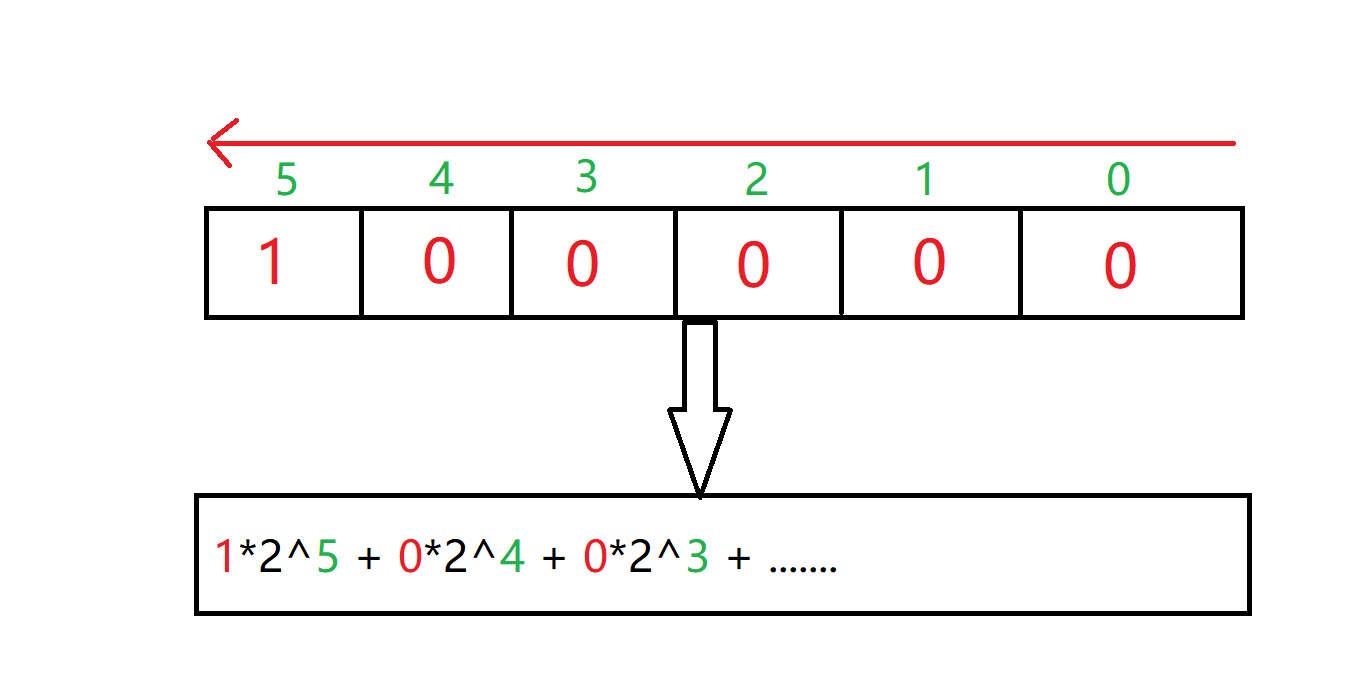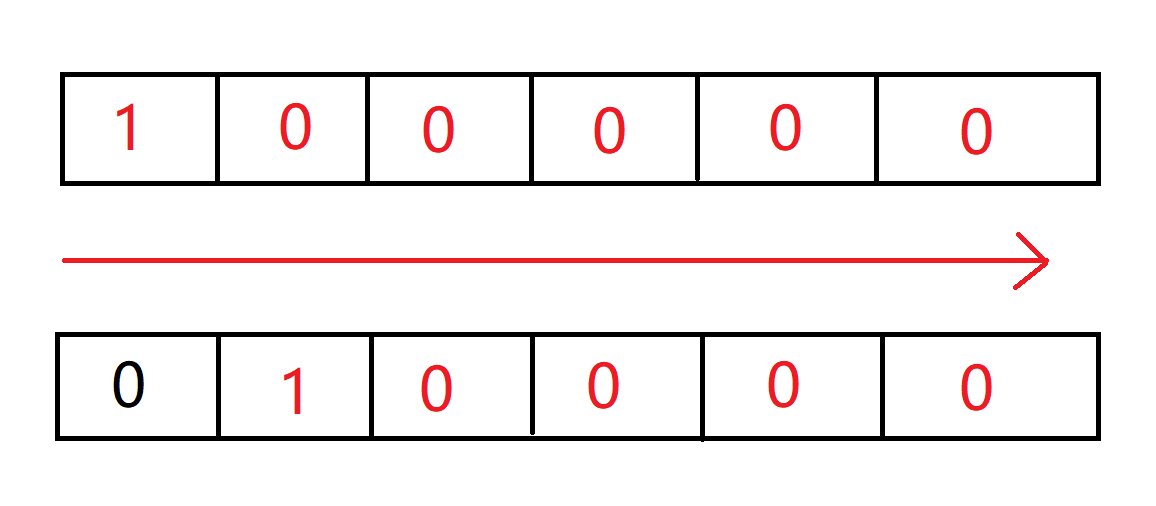（0 * 2 ^ 5）+（1 * 2 ^ 4）+（0 * 2 ^ 3）+（0 * 2 ^ 2）+（0 * 2 ^ 1）+（0 * 2 ^ 0）= 0 + 16 + 0 + 0 + 0 + 0 = 16。

 System.out.println( 32 >> 1);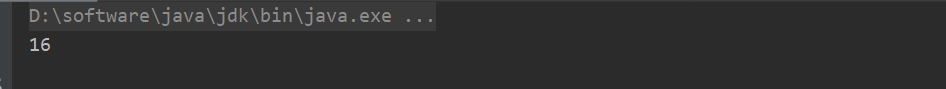### 添加指定索引元素分析

public static void main(String[] args) {

ArrayList<Integer> list = new ArrayList<>();

//添加元素2到索引5
}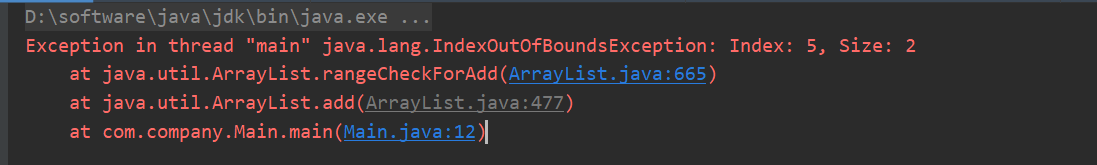//添加指定索引元素
public void add(int index, E element) {

//检查索引范围，确认是否添加

ensureCapacityInternal(size + 1);
System.arraycopy(elementData, index, elementData, index + 1,
size - index);
elementData[index] = element;
size++;
}
private void rangeCheckForAdd(int index) {

//要添加的元素索引不能大于数组实际大小或小于0，否则抛出异常
if (index > size || index < 0)
throw new IndexOutOfBoundsException(outOfBoundsMsg(index));
}

public static void main(String[] args) {
ArrayList<Integer> list = new ArrayList<>();
}

### trimToSize分析

public static void main(String[] args) {
ArrayList<Integer> list = new ArrayList<>(20);

list.trimToSize();
}

public void trimToSize() {
modCount++;
//若数组实际大小小于数组容量时
if (size < elementData.length) {
//若数组实际大小为0时则数组为空实例，否则复制数组到当前数组大小
elementData = (size == 0)
? EMPTY_ELEMENTDATA
: Arrays.copyOf(elementData, size);
}
}

### remove分析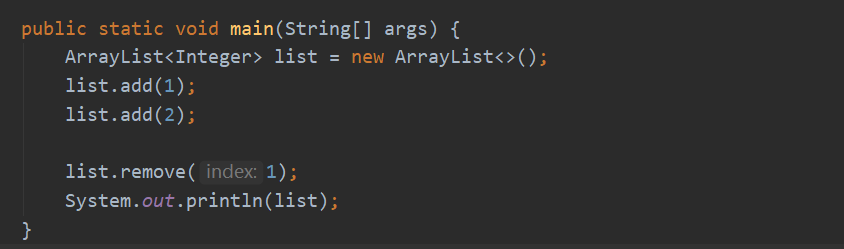//删除指定索引元素并返回删除元素值
public E remove(int index) {
//判断索引是否小于数组实际大小，否则抛出异常
rangeCheck(index);

modCount++;

//获取索引元素
E oldValue = elementData(index);

//获取复制数组时要复制元素个数
int numMoved = size - index - 1;
if (numMoved > 0)
System.arraycopy(elementData, index+1, elementData, index,
numMoved);

//将被删除的元素置为空，便于垃圾收集器回收
elementData[--size] = null;

return oldValue;
}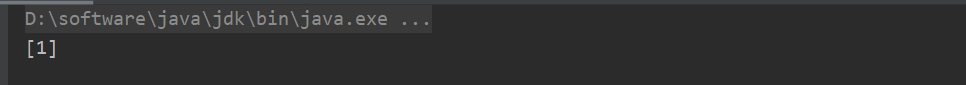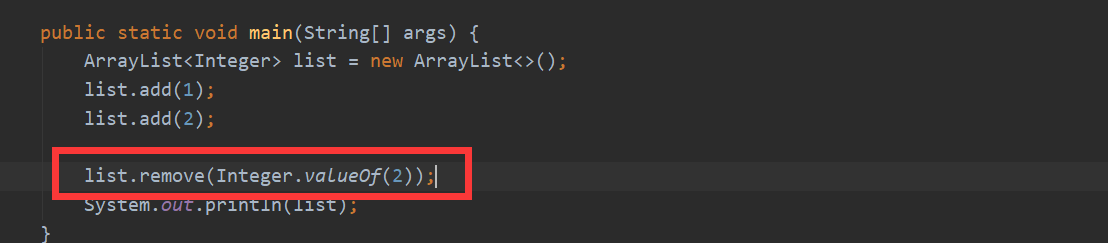//删除指定元素
public boolean remove(Object o) {
//若对象为空
if (o == null) {
//遍历数组
for (int index = 0; index < size; index++)
//找出数组中为空的元素
if (elementData[index] == null) {
//找出元素所在索引快速删除
fastRemove(index);
//返回删除成功
return true;
}
} else {
//若对象不为空
for (int index = 0; index < size; index++)
//找出数组中满足条件的元素
if (o.equals(elementData[index])) {
//找出元素所在索引快速删除
fastRemove(index);
//返回删除成功
return true;
}
}
//返回删除失败
return false;
}

//快速删除（本质上采用复制的方式）
private void fastRemove(int index) {

modCount++;
int numMoved = size - index - 1;
if (numMoved > 0)
System.arraycopy(elementData, index+1, elementData, index,
numMoved);
elementData[--size] = null;
}

public static void main(String[] args) {
ArrayList<Integer> list = new ArrayList<>();

Integer[] array = list.toArray(new Integer);

System.arraycopy(array, 3, array, 2,
3);

for (int i = 0; i < array.length; i++) {
System.out.println(array[i]);
}
}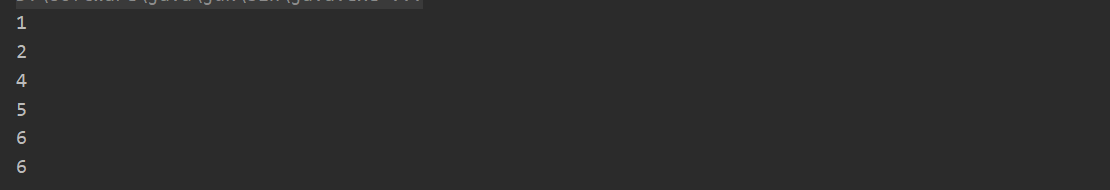## 总结

posted @ 2019-09-03 00:13  Jeffcky  阅读(353)  评论(2编辑  收藏  举报